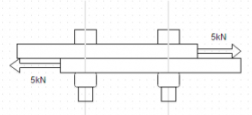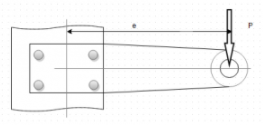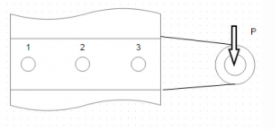Courses

# Test: Eccentric Loaded Bolted Joints

## 10 Questions MCQ Test Machine Design | Test: Eccentric Loaded Bolted Joints

Description
This mock test of Test: Eccentric Loaded Bolted Joints for Mechanical Engineering helps you for every Mechanical Engineering entrance exam. This contains 10 Multiple Choice Questions for Mechanical Engineering Test: Eccentric Loaded Bolted Joints (mcq) to study with solutions a complete question bank. The solved questions answers in this Test: Eccentric Loaded Bolted Joints quiz give you a good mix of easy questions and tough questions. Mechanical Engineering students definitely take this Test: Eccentric Loaded Bolted Joints exercise for a better result in the exam. You can find other Test: Eccentric Loaded Bolted Joints extra questions, long questions & short questions for Mechanical Engineering on EduRev as well by searching above.
QUESTION: 1

### If core diameter of bolt is 13.8cm the it’s nominal diameter is given by?Solution:

Explanation: D=d/0.8.

QUESTION: 2

### Refer to fig Two plates are fastened by means of two bolts. The yield strength of bolt is 400N/mm² and factor of safety is 4. Determine the permissible shear stress in the bolts.Solution:

Explanation: Permissible hear stress=0.5 x 400 /4 =50N/mm².

QUESTION: 3

### Refer to fig Two plates are fastened by means of two bolts. The yield strength of bolt is 400N/mm² and factor of safety is 4. Determine the size of the bolts.Solution:

Explanation: Permissible hear stress=0.5 x 400 /4 =50N/mm². P=2 (π xd²/4) x τ or 5000=2 x π x d² x 50/4 or d=7.97mm.

QUESTION: 4

The structure shown is subjected to an eccentric force P=5kN and eccentricity=500mm. The horizontal distance between two bolts is 200mm and vertical distance between bolts is 150mm. The yield strength of bolts is 400N/mm² and factor of safety is 3.Referring to fig , Determine the primary shear force.

Solution:

Explanation: Primary shear force P₁=P₂=P₃=P₄=P/4 =1250N.

QUESTION: 5

The structure shown is subjected to an eccentric force P=5kN and eccentricity=500mm. The horizontal distance between two bolts is 200mm and vertical distance between bolts is 150mm. The yield strength of bolts is 400N/mm² and factor of safety is 3.Referring to fig , Determine the secondary shear force.

Solution:

Explanation: Secondary shear force=Moment about CG x distance from CG/sum of squares of distance of bolts from CG. F= (Pe)xr₁/(r₁²+r₂²+r₃²+r₄²). Here r₁=r₂=r₃=r₄=125mm hence F= 5000 x 500/(4×125) or F=5000N.

QUESTION: 6

The structure shown is subjected to an eccentric force P=5kN and eccentricity=500mm. The horizontal distance between two bolts is 200mm and vertical distance between bolts is 150mm. The yield strength of bolts is 400N/mm² and factor of safety is 3.Referring to figure , determine the resultant shear force on the bolt lying left and above the CG.

Solution:

Explanation: After breaking shear force into primary and secondary shear force, primary acts along the vertical positively and secondary acts at an angle of 180-36.87’ ACW from the vertical. Hence net shear force= √ [5000cos36.8-1250]²+ [5000sin36.8]² =4068.58N.

QUESTION: 7

The structure shown is subjected to an eccentric force P=5kN and eccentricity=500mm. The horizontal distance between two bolts is 200mm and vertical distance between bolts is 150mm. The yield strength of bolts is 400N/mm² and factor of safety is 3.In figure , determine the resultant shear force on the bolt lying right and above the CG.

Solution:

Explanation: After breaking net shear force into primary and secondary shear force, primary acts along the vertical positively and secondary acts at an angle of 36.8’CW from the vertical. Hence net shear force = √ [5000cos36.8+1250]²+ [5000sin36.8]².

QUESTION: 8

The structure shown is subjected to an eccentric force P=5kN and eccentricity=500mm. The horizontal distance between two bolts is 200mm and vertical distance between bolts is 150mm. The yield strength of bolts is 400N/mm² and factor of safety is 3.In figure , determine the size of the bolts.

Solution:

Explanation: The maximum shear force to which any bolt is subjected is 6047.44N. Hence 0.5 x 400/3= 4 x 6047.44/πd² or d=10.74mm.

QUESTION: 9Which bolt is under maximum shear stress?

Solution:

Explanation: Primary shear force acts equally on the three bolts in the vertically upward direction while the moment is CW along CG so its effect on bolts will be ACW. Hence secondary shear force acts vertically upward on bolt 3 and vertically downward on bolt 1.

QUESTION: 10Arrange the bolts in order of decreasing shear stresses.

Solution:

Explanation: On bolt 3,primary and secondary shear stress act in same direction, on bolt 2 there is no secondary shear stress and on bolt 1 the two act in opposite direction.